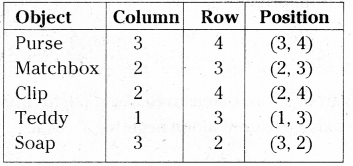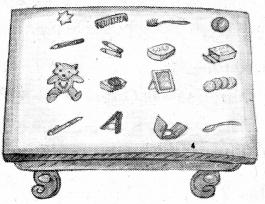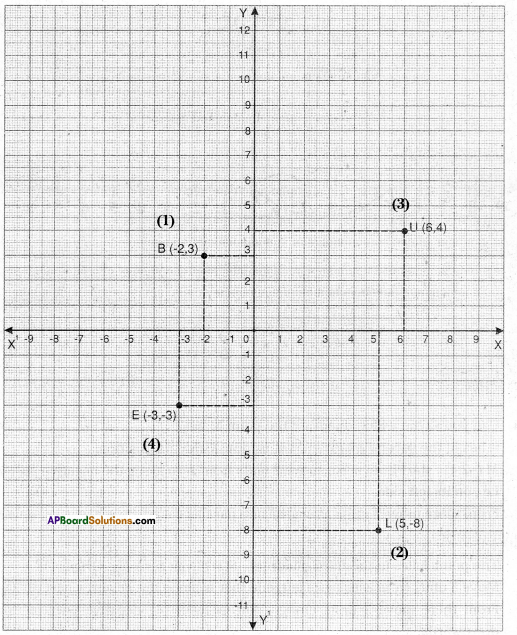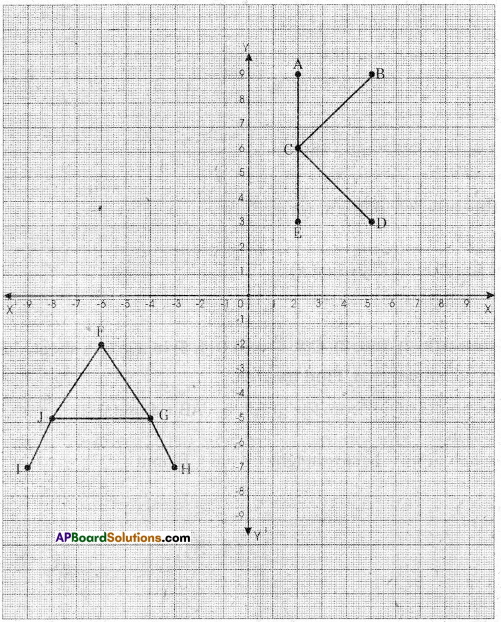# AP Board 9th Class Maths Solutions Chapter 5 Co-Ordinate Geometry InText Questions

AP State Syllabus AP Board 9th Class Maths Solutions Chapter 5 Co-Ordinate Geometry InText Questions and Answers.

## AP State Syllabus 9th Class Maths Solutions 5th Lesson Co-Ordinate Geometry InText Questions

Do This

Question
Describe the seating position of any five students in your classroom. [Page No. 108]
Solution:
Oral answer – depend on the classroom.Activity (Ring Game)

Question
Have you seen ‘Ring game’ in exhibitions ? We throw rings on the objects arranged in rows and columns. Observe the following picture. [Page No. 108]
Complete the following table.
Solution:Question
Is the object 3rd column and 4th row is same as 4th column 3rd row ?
Solution:
No

Do This

Question
Among the points given below, some of the points lie on X – axis. Identify them.
i) (0, 5) ii) (0, 0) iii) (3, 0) iv) (- 5, 0) v) (- 2, – 3) vi) (- 6,0) vii)(0,6) viii)(0, a) ix) (b, 0) [Page No. 114]
Solution:
The points (ii) (0, 0), (iii) (3, 0) (iv) (- 5, 0) (vi) (- 6, 0) and (ix) (b, 0) lie on X – axis.

Try These

Question 1.
Which axis the points such as (0, x), (0, y), (0, 2) and (0, – 5) lie on ? Why ? [Page No. 114]
Solution:
All the above points lie on Y – axis, since the X – coordinate of all these points is zero.

Question 2.
What is the general form of the points which lie on X – axis. [Page No. 114]
Solution:
The general form of points lying on X – axis is (x, 0).Do This

Question
Plot the following points on a Cartesian plane.
1. B (- 2, 3) 2. L (5, – 8) 3. U (6, 4) 4. E (-3, – 3) [Page No. 120]
Solution:Do This

Question
i) Write the co-ordinates of the points A, B, C, D and E. [Page No. 121]
ii) Write the co-ordinates of F, G, H, I and J.Solution”
i) A(2, 9) ; B(5, 9); C(2, 6) ; D(5, 3) ; E(2, 3)
ii) F(- 6, – 2) ; G(- 4, – 5) ; H(- 3, – 7) ; I(- 9, – 7) ; J(- 8, – 5)Activity

Question
Study the positions of different cities like Hyderabad, New Delhi, Chennai and Vishakapatnam with respect to longitudes and latitudes on a globe. [Page No. 123]
Solution:
Student ActivityCreative Activity

Question
Take a graph sheet and plot the following pairs of points on the axes and join them with line segments. [Page No. 123]
(1, 0) (0, 9) ; (2, 0) (0, 8) ; (3, 0) (0, 7) ; (4, 0) (0, 6) ;
(5, 0) (0, 5) ; (6, 0) (0, 4) ; (7, 0) (0, 3) ; (8, 0) (0, 2) ; (9, 0) (0, 1).
Try to complete the picture by using above points. What did you observe ?
Solution:
Student Activity

AP Board 9th Class Maths Solutions